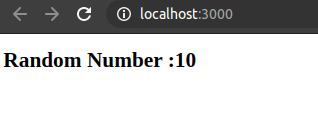# How to change state continuously after a certain amount of time in React?

• Last Updated : 01 Sep, 2020

To change a state continuously after a certain amount of time is required in a few cases for the toggling. First, make a function that is responsible for changing the state of the component. Then call the function from the constructor method for the first time.  Use the set interval method inside the function to change the state after a fixed amount of time. setInterval method takes two parameter callback and time. The callback function is called again and again after that given amount of time.  Use the setState method to change the state of the component.

```timing(){
setInterval(() => {
this.setState({
stateName : new-state-value
})
}, time)
}
```

Example 1: This example illustrates how to change the state continuously after a certain amount of time.

index.js:

## Javascript

 `import React from ``'react'` `import ReactDOM from ``'react-dom'` `import App from ``'./App'` ` `  `ReactDOM.render(, document.querySelector(``'#root'``))`

App.js:

## Javascript

 `import React, { Component } from ``'react'` ` `  `class App extends Component { ` `  ``constructor(props){ ` `    ``super``(props) ` `    ``this``.state = {Number : 0} ` `    ``this``.makeTimer() ` `  ``} ` ` `  `  ``makeTimer(){ ` `    ``setInterval(() => { ` `      ``let rand = Math.floor(Math.random() * 10) + 1 ` `        ``this``.setState({number: rand}) ` `    ``}, 1000) ` `  ``} ` `  ``render(){ ` `    ``return` `( ` `      ``
` `        ``

` `          ``Random Number :  ` `          ``{``this``.state.number} ` `        ``

` `      ``
` `    ``) ` `  ``} ` `} ` ` `  `export ``default` `App`

Output:My Personal Notes arrow_drop_up
Recommended Articles
Page :